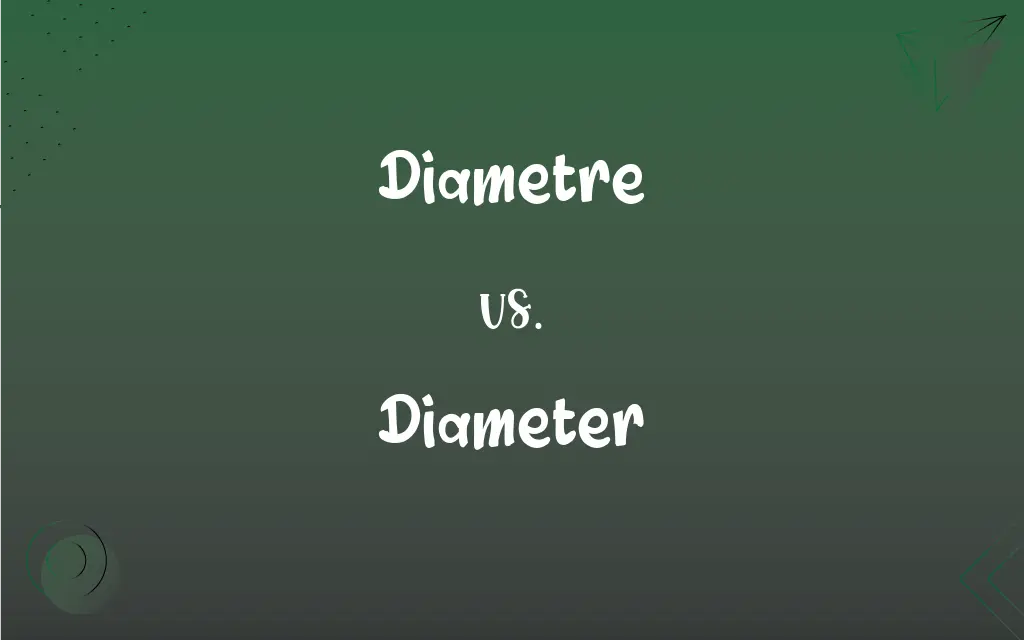# Diametre vs. Diameter: What's the Difference?## Diametre and Diameter Definitions

#### Diametre

Alternative form of diameter

#### Diameter

A straight line segment passing through the center of a figure, especially of a circle or sphere, and terminating at the periphery.

#### Diameter

The length of such a segment.

#### Diameter

Thickness or width.

#### Diameter

A unit of magnification equal to the number of times an object's linear dimensions is increased by the magnifying apparatus.

#### Diameter

(geometry) Any straight line between two points on the circumference of a circle that passes through the centre/center of the circle.

#### Diameter

(geometry) The length of such a line.

#### Diameter

(geometry) The maximum distance between any two points in a metric space

#### Diameter

(graph theory) The maximum eccentricity over all vertices in a graph.

#### Diameter

Any right line passing through the center of a figure or body, as a circle, conic section, sphere, cube, etc., and terminated by the opposite boundaries; a straight line which bisects a system of parallel chords drawn in a curve.

#### Diameter

The length of a straight line through the center of an object from side to side; width; thickness; as, the diameter of a tree or rock.

#### Diameter

The distance through the lower part of the shaft of a column, used as a standard measure for all parts of the order. See Module.

#### Diameter

The length of a straight line passing through the center of a circle and connecting two points on the circumference

#### Diameter

A straight line connecting the center of a circle with two points on its perimeter (or the center of a sphere with two points on its surface)